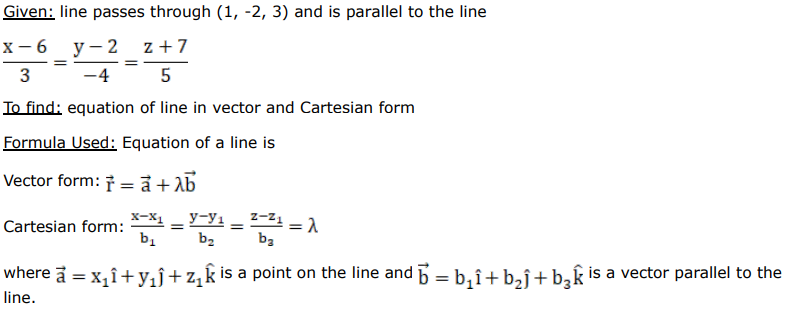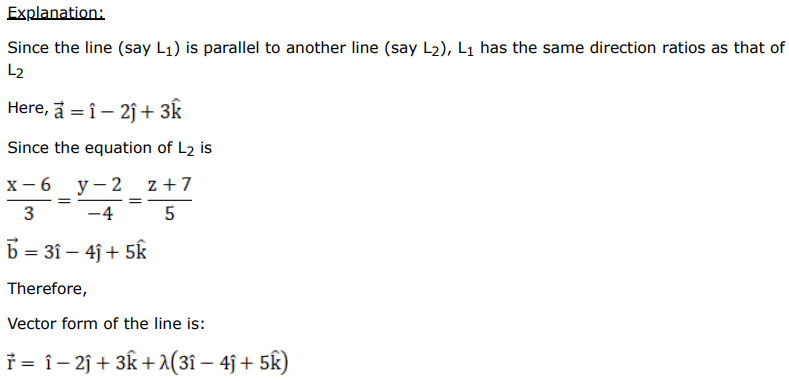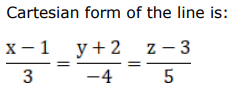# Find the equations of the line passing through the pointQuestion:

Find the equations of the line passing through the point $(1,-2,3)$ and parallel to the line $\frac{x-6}{3}=\frac{y-2}{-4}=\frac{z+7}{5}$. Also find the vector form of this equation so obtained.

Solution: